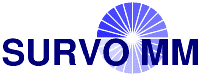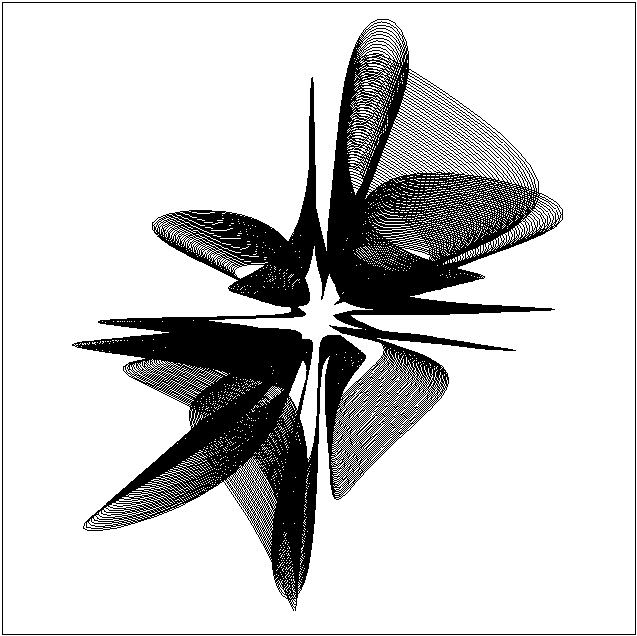# Environment for creative processing of text and numerical data## Spiral

S.Mustonen (1976), Survo Users' Guide (1992), p. 332### Edit field:

```   1 *
4 *`PLOT X(T)=0.03*T*(exp(-1+sin(17*T)))*cos(T),`
5 *     `Y(T)=0.09*T*(exp(-1.9+cos(11*T)))*sin(T+0.5)`